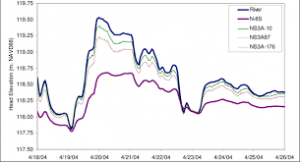## How to Calculate and Solve for Elevation Correction | GravityThe image above represents elevation correction.

To compute for elevation correction, two essential parameters are needed and these parameters are Free Air Correction (δgFand Bouguer Correction (δgB).

The formula for calculating elevation correction:

δgE = δgF – δgB

Where:

δgE = Elevation Correction
δgF = Free Air Correction
δgB = Bouguer Correction

Let’s solve an example;
Find the elevation correction when the free air correction is 12 and the bourger correction is 17.

This implies that;

δgF = Free Air Correction = 12
δgB = Bouguer Correction = 17

δgE = δgF – δgB
δgE = 12 – 17
δgE = -5

Therefore, the elevation correction is -5 mGal.

Calculating the Free Air Correction when the Elevation Correction and the Bourger Correction is Given.

δgF = δgE + δgB

Where;

δgF = Free Air Correction
δgE = Elevation Correction
δgB = Bouguer Correction

Let’s solve an example;
Find the free air correction when the elevation correction is 34 and the bourger correction is 21.

This implies that;

δgE = Elevation Correction = 34
δgB = Bouguer Correction = 21

δgF = δgE + δgB
δgF = 34 + 21
δgF = 55

Therefore, the free air correction is 55.